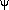### BCD Statistics Topics in Using R for Statistics and Data Analysis - under development Tutorials for APSY510/511

Last updated 8-6-2020

Basics of R UsageIntroduction, Installation, Maintenance of R, RStudio, and R packages (HTML) (PDF)Importing, managing and saving data in R (HTML) (PDF)Data Subsetting: Selecting Cases and/or Variables, etc (HTML) (PDF)
Linear Regression and CorrelationBivariate Correlation and Simple Regression (HTML) (PDF)Scatterplots (HTML) (PDF)Basics of Linear Modeling with multiple IVs (Multiple Regression) R (HTML) (PDF) (ePub)
Experimental Design MethodsOne Factor Between Group ANOVA (HTML) (PDF) (ePub)One Factor Repeated Measures ANOVA (HTML) (PDF) (ePub)Two Factor Between Group ANOVA (HTML) (PDF)Introduction to Confirmatory Factor Analysis in R (HTML) (PDF) (ePub)
Miscl TopicsWide-Long Data Reshaping (HTML) (PDF)Power, Effect Size, Sample Size Choice and an introduction to non-central distributions (HTML) (PDF)